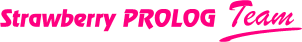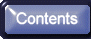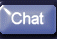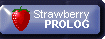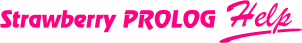Define functions and Built-in predicates

In Strawberry Prolog you have the unique possibility to define your own functions and built-in predicates. Here you can normally ask the question: What is the difference between standard Prolog predicates and built-in predicates. Usually in the most Prolog compilers built-in predicates are called to such predicates you cannot define alone and that is why they are defined in the system. In Strawberry Prolog the situation is different, you can define your own built-in predicates. So what is the difference between built-in and standard predicates? You define built-in predicates with fixed types and fixed number of the arguments. When these predicates are executed then the types and the number of the arguments are checked and if it is not correct then an error message is displayed. Also, in the built-in predicates you can give an expression as an argument. This expression will be calculated before execution of the built-in predicate, then the predicate will be executed and the value of the expression will be given to it on the place of respective argument.

Maybe you are a little bit disappointed by such built-in predicates because you expected that they are written in another language like C and also that they are hidden somewhere in the system and you cannot see their definition. It doesn't matter that your predicate is built-in or not, if you want to make it written in C or in another language then you can do this and put it in a DLL file or in OLE server. After that you can call such a function from Strawberry Prolog. If you want to hide your predicate's definition then you can put it in Standard.add file or you can create your own ADD file to contain your built-in predicates and other often used by you Prolog source.

Well, but how to define functions and Built-in predicates in Strawberry Prolog. Here is how such definitions look like:

R is f(X1, X2, ... , Xn) :- G1, G2, ... , Gm.
*** p(X1, X2, ... , Xn) :- G1, G2, ... , Gm.

Where Xi is variable with some specifiers eventually. Here is the list of all possible types specifiers which you can use in the definition of functions and of built-in predicates:

int X - integer
float X - floating point number
str X - string
obj(X) - object
var(X) - variable
term(X) - term, i.e. no one from the above
io(X) - used for input and output
num(X) - the same as int float X
X - the same as int float str obj(var(term(X))) , i.e. calculate and don't check the type
nocalc(X) - this means that this argument should not be calculated and will be given as a term as this is with the standard Prolog predicates.

The first three specifiers are defined as prefix operators and that's why they don't need brackets. You can define one argument using several specifiers. In this case the meaning is OR, i.e. the value must be one of the specified types. The row and repetition of specifiers are not important, i.e int float X is the same as float int X or even int int float X . You cannot combine the specifier nocalc with any of the rest.

As you can see the definition of built-in predicate is the same as the definition of the standard one with the difference that you have to put the key word *** and the arguments cannot be any terms like this is in the standard predicates but they must be only variables, eventually with some specifiers. You can define one built-in predicate (or function) with more than one clause as with standard predicates but in this case in all definition clauses the number of variables (in the head) and all specifiers must be the same. For example:

*** p(X1, X2, ... , Xn) :- body_1.
.
.
.
*** p(X1, X2, ... , Xn) :- body_m.

Here if the first body fails the compiler will try to satisfy the next one and so on.

Let's see an example for defining of function and for built-in predicate:

R is add(X, Y) :- R:=X+Y.
*** my_write(X) :- beep, writeq(X), nl.

What we have defined? The new function add is actually the same as the + function which we already have. You can put it in expressions and it will calculate the expressions X and Y and after that will execute the body of the function where it will calculate the value of R. The only small difference between add and + is that the first will accept any kind of expressions for X and Y with the difference of + which accepts only integer, float and string values for them. This means that you can give two variables or terms for X and Y and add will accept them. Anyway, when the execution of the body of add starts you will receive an error message from the function + . This message will say that the arguments of + cannot be variables or terms but you will not get such a message for the function add .

If you want then you can make a type check in the add function. Let's say that we want to check whether their arguments are integer or float. Then we can define add as follows:

R is add(int float X, int float Y) :- R:=X+Y.

Now our add function is like + but only for integer and float numbers. If we try to concatenate strings with it then we will receive an error message which says that the arguments can not be strings.

The built-in predicate my_write is the same as write predicate (only one beep and one new line are small differences). If we call my_write(2+2) in our program then what will be written in the Output window? Will it be 4 as if we call write or will it be 2+2 as if we call writeq . The predicate my_write will write 4 although we use writeq in its body because the expression will be calculated before the execution of the body. If we want to make my_write like writeq then we should define it as follows:

*** my_write(nocalc(X)) :- beep, writeq(X), nl.

When defining functions and built-in predicates you can use also the operator :-- instead of :- . What is the difference? After the compilation all clauses which have the same heads are represented as a chain. Every one has a pointer to the next. So, when the program is executed if the first clause from the chain fails then the interpreter takes the next one. If you define a clause using :-- instead of :- then this clause will have a chain pointer to itself. This mean that this clause will be recalled forever (the same as if this clause was infinitely many times in the program). For example by :-- is defined the predicate repeat.

For examples of functions defined on Prolog is a good idea to look at Strawberry functions . Their definitions are in the Standard.add file.